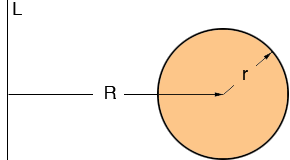SEARCH HOMEMath Central Quandaries & QueriesSubject: Math - calculating volume Name: Bubba Who are you: Student For a science project, I'm collecting methane gas in an inner tube. In addition to measuring psi of the inner tube, I'd like to calculate the volume of gas collected. What is the formula for or how would I calculate the volume of an inner tube? I appreciate any help you can give me. Thanks so much. BubbaHi Bubba,

The shape of an inner tube is a torus. You can form a torus by rotating a circle of radius r around a line L which is R units from the centre of the circle.With these variables the volume or the torus on Math World. It is

V = 2 π2 R r2

PennyMath Central is supported by the University of Regina and The Pacific Institute for the Mathematical Sciences.